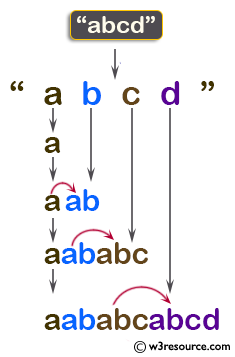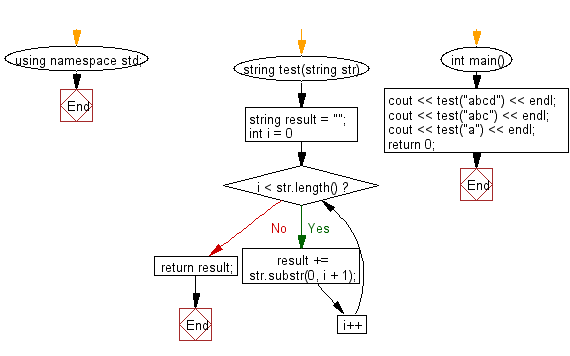﻿ C++ : Create a string like aababcabcd from a string abcd# C++ Exercises: Create a string like 'aababcabcd' from a given string 'abcd'

## C++ Basic Algorithm: Exercise-29 with Solution

Write a C++ program to create a string like "aababcabcd" from a given string "abcd".

Sample Solution:

C++ Code :

``````#include <iostream>
using namespace std;

string test(string str)
{
string result = "";
for (int i = 0; i < str.length(); i++)
{
result += str.substr(0, i + 1);
}
return result;
}

int main()
{
cout << test("abcd") << endl;
cout << test("abc") << endl;
cout << test("a") << endl;
return 0;
}
``````

Sample Output:

```aababcabcd
aababc
a
```

Pictorial Presentation:Flowchart:C++ Code Editor: